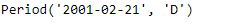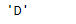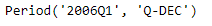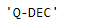# Python | Pandas Period.freqstr

• Last Updated : 06 Jan, 2019

Python is a great language for doing data analysis, primarily because of the fantastic ecosystem of data-centric python packages. Pandas is one of those packages and makes importing and analyzing data much easier.

Pandas` Period.freqstr` attribute returns the string alias of the Time series frequency applied on the given Period object.

Syntax : Period.freqstr

Parameters : None

Return : string

Example #1: Use `Period.freqstr` attribute to find the string alias of the time series frequency applied on the given Period object.

 `# importing pandas as pd``import` `pandas as pd`` ` `# Create the Period object``prd ``=` `pd.Period(freq ``=``'D'``, year ``=` `2001``, month ``=` `2``, day ``=` `21``)`` ` `# Print the Period object``print``(prd)`

Output :Now we will use the `Period.freqstr` attribute to find the string alias of the frequency applied on prd object.

 `# return the string alias of the frequency``prd.freqstr`

Output :As we can see in the output, the `Period.freqstr `attribute has returned ‘D’ indicating that the time series frequency applied on the given object was day.

Example #2: Use `Period.freqstr` attribute to find the string alias of the time series frequency applied on the given Period object.

 `# importing pandas as pd``import` `pandas as pd`` ` `# Create the Period object``prd ``=` `pd.Period(freq ``=``'Q'``, year ``=` `2006``, quarter ``=` `1``)`` ` `# Print the object``print``(prd)`

Output :Now we will use the `Period.freqstr` attribute to find the string alias of the frequency applied on prd object.

 `# return the string alias of the frequency``prd.freqstr`

Output :As we can see in the output, the `Period.freqstr `attribute has returned ‘Q-DEC’ indicating that the time series frequency applied on the given object was the end quarter of the year.

My Personal Notes arrow_drop_up